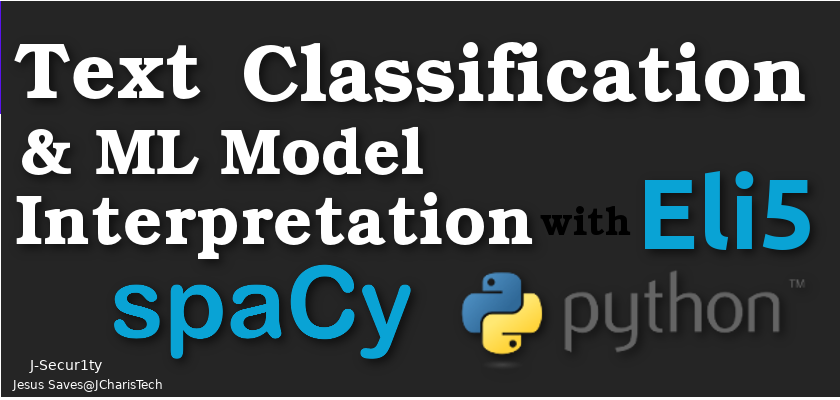# Text Classification and ML Model Interpretation with ELi5,Sklearn and SpaCy

In this tutorial we will see how to classify text/document using machine learning and then move on to interpret our classification model with Eli5.

This is the workflow we will be using in this project.

• Text Preprocessing
• We will be using spacy and basic python to preprocess our documents to get a clean dataset
• We will remove all stop words and build a tokenizer and a couple of lemmas.
• This is to help improve our dataset which we will feed into our model.

• Text Classification and Model Building
• Since we are working with text document we will be using countvectorizer or TFID to help in our vectorization
• Vectorization here means we are converting our text into numbers so that our ML will be able to understand it.
• We will then build our model using algorithms from scikitlearn package
• You can choose any algorithm as you like.

• Prediction
• We will then use our trained model to do some predictions
• We calculate the accuracy of our model

• Interpretation of Our Machine Learning Model
• The next step involves using Eli5 to help interpret and explain why our ML model gave us such a prediction
• We will then check for how trustworthy our interpretation is with Eli5’s metrics

Let us check the code

In :

```# load EDA Pkgs
import pandas as pd
import numpy as np
```
In :
```# Load NLP pkgs
import spacy
```
In :
```from spacy.lang.en.stop_words import STOP_WORDS
```
In :
```# Use the punctuations of string module
import string
punctuations = string.punctuation
```
In :
```# Creating a Spacy Parser
from spacy.lang.en import English
parser = English()
```
In :
```# Build a list of stopwords to use to filter
stopwords = list(STOP_WORDS)
```
In :
```stopwords

```
In :
```def spacy_tokenizer(sentence):
mytokens = parser(sentence)
mytokens = [ word.lemma_.lower().strip() if word.lemma_ != "-PRON-" else word.lower_ for word in mytokens ]
mytokens = [ word for word in mytokens if word not in stopwords and word not in punctuations ]
return mytokens
```
In :
```ex1 = "He was walking with the walker in the Wall he may had sat and run with the runner"
```
In :
```spacy_tokenizer(ex1)
```
Out:
`['walk', 'walker', 'wall', 'sit', 'run', 'runner']`
In :
```# Load ML Pkgs
# ML Packages
from sklearn.feature_extraction.text import CountVectorizer,TfidfVectorizer
from sklearn.metrics import accuracy_score
from sklearn.base import TransformerMixin
from sklearn.pipeline import Pipeline
from sklearn.svm import LinearSVC
from sklearn.svm import SVC
```
In :
```# Load Interpretation Pkgs
import eli5
```
In :
```# Load dataset
```
In :
```df.head()
```
In :
```df.shape
```
Out:
`(2745, 4)`
In :
```df.columns
```
Out:
`Index(['Unnamed: 0', 'Unnamed: 1', 'Message', 'Target'], dtype='object')`
In :
```#Custom transformer using spaCy
class predictors(TransformerMixin):
def transform(self, X, **transform_params):
return [clean_text(text) for text in X]
def fit(self, X, y=None, **fit_params):
return self
def get_params(self, deep=True):
return {}
```
In :
```# Basic function to clean the text
def clean_text(text):
return text.strip().lower()
```
In :
```# Vectorization
vectorizer = CountVectorizer(tokenizer = spacy_tokenizer, ngram_range=(1,1))
# classifier = LinearSVC()
classifier = SVC(C=150, gamma=2e-2, probability=True)
```
In :
```# Using Tfidf
tfvectorizer = TfidfVectorizer(tokenizer = spacy_tokenizer)
```
In :
```# Splitting Data Set
from sklearn.model_selection import train_test_split
```
In :
```# Features and Labels
X = df['Message']
ylabels = df['Target']
```
In :
```X_train, X_test, y_train, y_test = train_test_split(X, ylabels, test_size=0.2, random_state=42)
```
In :
```X_train
```
In :
```X_train.shape
```
Out:
`(2196,)`
In :
```# Create the  pipeline to clean, tokenize, vectorize, and classify
pipe = Pipeline([("cleaner", predictors()),
('vectorizer', vectorizer),
('classifier', classifier)])
```
In :
```# Fit our data
pipe.fit(X_train,y_train)
```
Out:
```Pipeline(memory=None,
steps=[('cleaner', <__main__.predictors object at 0x000002CEDE98C9B0>), ('vectorizer', CountVectorizer(analyzer='word', binary=False, decode_error='strict',
dtype=<class 'numpy.int64'>, encoding='utf-8', input='content',
lowercase=True, max_df=1.0, max_features=None, min_df=1,
...',
max_iter=-1, probability=True, random_state=None, shrinking=True,
tol=0.001, verbose=False))])```
In :
```X_test.shape
```
Out:
`(549,)`
In :
```X_test.values
```
Out:
`'It is a true classic.  '`
In :
```# Predicting with a test dataset
sample_prediction = pipe.predict(X_test)

```
In :
```print("Accuracy Score:",pipe.score(X_test, y_test))
```
```Accuracy Score: 0.7540983606557377
```
In :
```# Prediction Results
# 1 = Positive review
# 0 = Negative review
for (sample,pred) in zip(X_test,sample_prediction):
print(sample,"Prediction=>",pred)
```
```Great pork sandwich. Prediction=> 1
It is a true classic.   Prediction=> 0
It's close to my house, it's low-key, non-fancy, affordable prices, good food. Prediction=> 0
Audio Quality is poor, very poor. Prediction=> 0
We loved the biscuits!!! Prediction=> 1

```

### Interpreting Our Model

• Eli5
• Data
• Model
• Target Names
• Function
In :
```from eli5.lime import TextExplainer
```
In :
```pipe.predict_proba
```
Out:
`<function sklearn.pipeline.Pipeline.predict_proba(self, X)>`
In :
```exp = TextExplainer(random_state=42)
```
In :
```X_test.values
```
Out:
`'Great pork sandwich.'`
In :
```exp.fit(X_test.values, pipe.predict_proba)
```
Out:
```TextExplainer(char_based=False,
clf=SGDClassifier(alpha=0.001, average=False, class_weight=None,
early_stopping=False, epsilon=0.1, eta0=0.0, fit_intercept=True,
l1_ratio=0.15, learning_rate='optimal', loss='log', max_iter=None,
n_iter=None, n_iter_no_change=5, n_jobs=None, penalty='elasticnet',
power_t=0.5,
random_state=<mtrand.RandomState object at 0x000002CED2CC19D8>,
shuffle=True, tol=0.001, validation_fraction=0.1, verbose=0,
warm_start=False),
expand_factor=10, n_samples=5000, position_dependent=False,
random_state=42, rbf_sigma=None,
sampler_params=None, token_pattern='(?u)\\b\\w+\\b',
weights=array([0.7, 0.3])),
token_pattern='(?u)\\b\\w+\\b',
vec=CountVectorizer(analyzer='word', binary=False, decode_error='strict',
dtype=<class 'numpy.int64'>, encoding='utf-8', input='content',
lowercase=True, max_df=1.0, max_features=None, min_df=1,
ngram_range=(1, 2), preprocessor=None, stop_words=None,
strip_accents=None, token_pattern='(?u)\\b\\w+\\b', tokenizer=None,
vocabulary=None))```
In :
```ylabels.unique()
```
Out:
`array([0, 1], dtype=int64)`
In :
```target_names = ['Negative','Positive']
```
In :
```exp.show_prediction()
```
Out:

y=1 (probability 0.857, score 1.789) top features

Contribution? Feature
+1.859 Highlighted in text (sum)
-0.070 <BIAS>

great pork sandwich.

In :
```exp.show_prediction(target_names=target_names)
```
Out:

y=Positive (probability 0.857, score 1.789) top features

Contribution? Feature
+1.859 Highlighted in text (sum)
-0.070 <BIAS>

great pork sandwich.

In :
```exp.metrics_
```
Out:
```{'mean_KL_divergence': 0.0006893282060298322, 'score': 1.0}

```
In :
```exp.show_weights()
```
Out:

y=1 top features

Weight? Feature
+1.918 great
+0.144 great pork
+0.133 pork sandwich
-0.070 <BIAS>
-0.118 sandwich
-0.218 pork
In :
```# Check For Vectorizer Used
exp.vec_
```
Out:
```CountVectorizer(analyzer='word', binary=False, decode_error='strict',
dtype=<class 'numpy.int64'>, encoding='utf-8', input='content',
lowercase=True, max_df=1.0, max_features=None, min_df=1,
ngram_range=(1, 2), preprocessor=None, stop_words=None,
strip_accents=None, token_pattern='(?u)\\b\\w+\\b', tokenizer=None,
vocabulary=None)```
In :
```# Check For Classifer Used
exp.clf_
```
Out:
```SGDClassifier(alpha=0.001, average=False, class_weight=None,
early_stopping=False, epsilon=0.1, eta0=0.0, fit_intercept=True,
l1_ratio=0.15, learning_rate='optimal', loss='log', max_iter=None,
n_iter=None, n_iter_no_change=5, n_jobs=None, penalty='elasticnet',
power_t=0.5,
random_state=<mtrand.RandomState object at 0x000002CECAE63240>,
shuffle=True, tol=0.001, validation_fraction=0.1, verbose=0,
warm_start=False)```
` `

Below is a video tutorial of the entire process